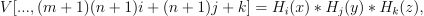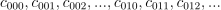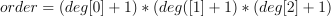/NumPy 1.17

# numpy.polynomial.hermite.hermvander3d

`numpy.polynomial.hermite.hermvander3d(x, y, z, deg)` [source]

Pseudo-Vandermonde matrix of given degrees.

Returns the pseudo-Vandermonde matrix of degrees `deg` and sample points `(x, y, z)`. If `l, m, n` are the given degrees in `x, y, z`, then The pseudo-Vandermonde matrix is defined bywhere `0 <= i <= l`, `0 <= j <= m`, and `0 <= j <= n`. The leading indices of `V` index the points `(x, y, z)` and the last index encodes the degrees of the Hermite polynomials.

If `V = hermvander3d(x, y, z, [xdeg, ydeg, zdeg])`, then the columns of `V` correspond to the elements of a 3-D coefficient array `c` of shape (xdeg + 1, ydeg + 1, zdeg + 1) in the orderand `np.dot(V, c.flat)` and `hermval3d(x, y, z, c)` will be the same up to roundoff. This equivalence is useful both for least squares fitting and for the evaluation of a large number of 3-D Hermite series of the same degrees and sample points.

Parameters: `x, y, z : array_like` Arrays of point coordinates, all of the same shape. The dtypes will be converted to either float64 or complex128 depending on whether any of the elements are complex. Scalars are converted to 1-D arrays. `deg : list of ints` List of maximum degrees of the form [x_deg, y_deg, z_deg]. `vander3d : ndarray` The shape of the returned matrix is `x.shape + (order,)`, where. The dtype will be the same as the converted `x`, `y`, and `z`.

#### Notes

New in version 1.7.0.